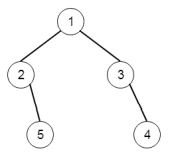# Program to find the left side view of a binary tree in C++

Suppose we have a binary tree, if we see the tree from left side, then we can see some elements of it. we have to display those elements. So if the tree is like −The output will be [1,2,5]

To solve this, we will follow these steps −

• Define an array ret

• Define a function dfs(), this will take node, c initialize it with 1,

• if node is null, then −

• return

• if c > lvl, then −

• lvl := c

• insert value of node into ret

• dfs(left of node, c + 1)

• dfs(right of node, c + 1)

• From the main method, do the following −

• lvl := -1

• dfs(root, 0)

• return ret

Let us see the following implementation to get better understanding −

## Example

Live Demo

#include <bits/stdc++.h>
using namespace std;
void print_vector(vector<int> v){
cout << "[";
for(int i = 0; i<v.size(); i++){
cout << v[i] << ", ";
}
cout << "]"<<endl;
}
class TreeNode{
public:
int val;
TreeNode *left, *right;
TreeNode(int data){
val = data;
left = right = NULL;
}
};
class Solution {
public:
vector <int> ret;
int lvl;
void dfs(TreeNode* node, int c = 1){
if(!node)
return;
if(c > lvl){
lvl = c;
ret.push_back(node->val);
}
dfs(node->left, c + 1);
dfs(node->right, c + 1);
}
vector<int> solve(TreeNode* root) {
lvl = -1;
dfs(root, 0);
return ret;
}
};
main(){
TreeNode *root = new TreeNode(1);
root->left = new TreeNode(2);
root->right = new TreeNode(3);
root->left->right = new TreeNode(5);
root->right->right = new TreeNode(4);
Solution ob;
print_vector(ob.solve(root));
}

## Input

TreeNode *root = new TreeNode(1);
root->left = new TreeNode(2);
root->right = new TreeNode(3);
root->left->right = new TreeNode(5);
root->right->right = new TreeNode(4);

## Output

[1,2,5]